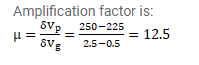# A triode value operates at Vp=225V and Vg= - 0.5V.

Question:

A triode value operates at $\mathrm{V}_{\mathrm{p}}=225 \mathrm{~V}$ and $\mathrm{V}_{\mathrm{g}}=-0.5 \mathrm{~V}$. The plate current remains unchanged if the plate voltage is increased to $250 \mathrm{~V}$ and the grid voltage is decreased to $-2.5 \mathrm{~V}$. Calculate the amplification factor.

Solution: